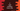# C program to read numbers from a file and store them in an array## C program to read numbers from a file and store them in an array:

In this post, we will learn how to read numbers from a text file and store them in an array in C. One text file is given and this file holds different number values. The C program will read all these values from that file and put them in an array. Also, it will print the array content.

### Create the text file:

For this example, create one file testfile.txt with the below text:

``````10
20
30
40
50``````

### C Program:

Below C program will read the numbers from the above text file and put them in an array. Create one example.c file in the same folder of testfile.txt with the below code:

``````#include <stdio.h>

int main(void)
{
int numbers;
int i = 0;
FILE *file;

if (file = fopen("testfile.txt", "r"))
{
while (fscanf(file, "%d", &numbers[i]) != EOF)
{
i++;
}
fclose(file);

numbers[i] = '\0';

for (i = 0; numbers[i] != '\0'; i++)
printf("%d\n", numbers[i]);
}

return 0;
}``````

Here,

• numbers is an array to hold the numbers
• file is the file pointer. This file pointer is used to hold the file reference once it is open.
• Using fopen, we are opening the file in read more. The r is used for read mode. If the file is opened using fopen, it scans the content of the file.
• The while loop reads the file and puts the content i.e. the numbers in the numbers array. The i is used to point to the current location in the array. It starts from 0 and it is incremented by 1 on each iteration.
• Once the while loop ends, we are closing the file using fclose. Also, as the reading is completed, we are adding \0 at the end of the array.
• The last for loop is used to print the contents of the numbers array, i.e. the numbers of the text file.

### Output:

It will print the contents of the file, i.e. the numbers.